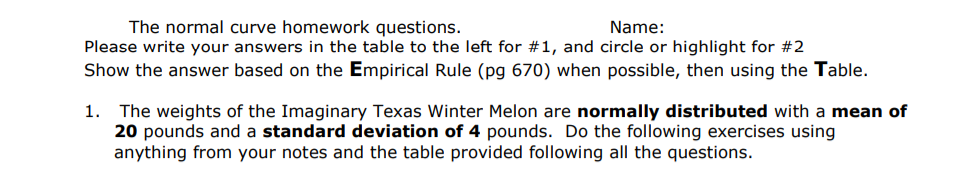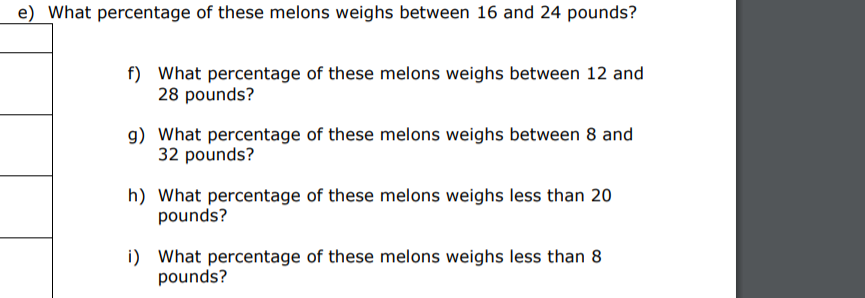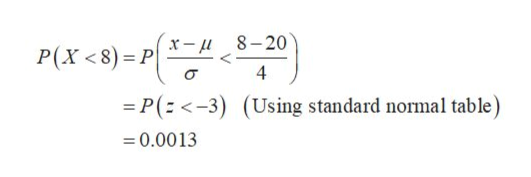# The normal curve homework questions.Please write your answers in the table to the left for #1, and circle or highlight for #2Show the answer based on the Empirical Rule (pg 670) when possible, then using the Table.Name:The weights of the Imaginary Texas Winter Melon are normally distributed with a mean of20 pounds and a standard deviation of 4 pounds. Do the following exercises usinganything from your notes and the table provided following all the questions.1. e) What percentage of these melons weighs between 16 and 24 pounds?f) What percentage of these melons weighs between 12 and28 pounds?g) What percentage of these melons weighs between 8 and32 pounds?h) What percentage of these melons weighs less than 20pounds?) What percentage of these melons weighs less than 8pounds?

Question
87 views

Can you please explain how to solve part i, using both the empirical rule and using a Z table?help_outlineImage TranscriptioncloseThe normal curve homework questions. Please write your answers in the table to the left for #1, and circle or highlight for #2 Show the answer based on the Empirical Rule (pg 670) when possible, then using the Table. Name: The weights of the Imaginary Texas Winter Melon are normally distributed with a mean of 20 pounds and a standard deviation of 4 pounds. Do the following exercises using anything from your notes and the table provided following all the questions. 1. fullscreenhelp_outlineImage Transcriptionclosee) What percentage of these melons weighs between 16 and 24 pounds? f) What percentage of these melons weighs between 12 and 28 pounds? g) What percentage of these melons weighs between 8 and 32 pounds? h) What percentage of these melons weighs less than 20 pounds? ) What percentage of these melons weighs less than 8 pounds? fullscreen
check_circle

Step 1

i) Consider X be a random variable which follows random variable indicates the weight of melons. The mean is 20 pounds with a standard deviation of 4 pounds.

The probability that these melons weighs less than 8 pounds is,help_outlineImage Transcriptionclose8-20 x-u P(X < 8) P 4 P(<-3) (Using standard normal table) 0.00 13 fullscreen
Step 2

Therefore, the required percentage is,

Percentage = Probab...

### Want to see the full answer?

See Solution

#### Want to see this answer and more?

Solutions are written by subject experts who are available 24/7. Questions are typically answered within 1 hour.*

See Solution
*Response times may vary by subject and question.
Tagged in

### Statistics